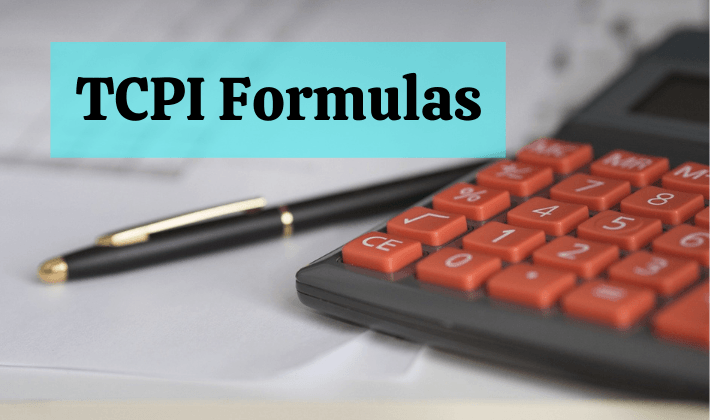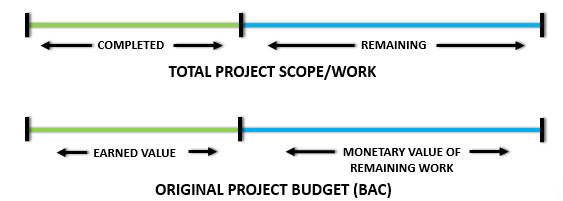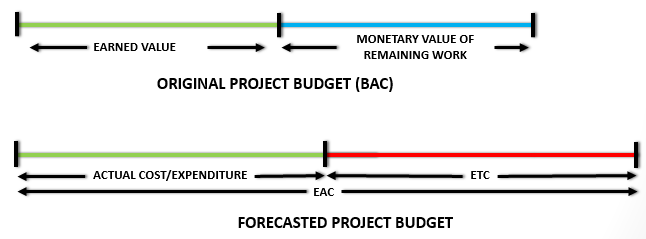To Complete Performance Index (TCPI) is the estimated cost efficiency required to complete the remaining project work within a defined budget.

The defined budget could be the original budget i.e., Budget At Completion (BAC) or it could be the revised budget Estimate At Completion (BAC).

TCPI is different from Cost Performance Index (CPI). TCPI is the future projected cost efficiency whereas CPI is the current efficiency of the project.

You will find detailed explanation of TCPI in this article. It includes:

1. TCPI definition and meaning in Project Management
2. Importance of TCPI for Earned Value Management (EVM)
3. TCPI formulas, examples, and calculations
4. Difference between BAC and EAC formulas
5. Difference between CPI and TCPI
6. strategies to solve mathematical questions for the PMP exam

You can also look at the following video to understand TCPI.

## To Complete Performance Index: Definition & Example

We already know that TCPI is the future projected cost efficiency to complete the project within a defined budget.

Let’s take a small example to understand the above definition. Let’s say that you are running a full marathon of 42 km.

Let’s suppose that you had set yourself a target of 4 hours to finish the race before starting. However, after running for 2 hours, you realize that you have completed only 18 km. This is less that 50% of the full distance.

Even though you are lagging behind, you still want to meet your original target of 4 hours. So, how will you do it?

You will, obviously, increase your speed to complete the race in the defined time limit.

Your current speed (running efficiency) till now is 9 km/hr (18 divided by 2). In order to complete the race in pre-defined time-limit, you will have to increase your future speed. You will have to cover the remaining distance (24 km) in the remaining time (2 km) i.e. you will have to increase your speed to 12 km/hr (24 divided by 2).

TCPI is very similar to the above example. Let us take a look at a EVM scenario.

## Importance of TCPI in Project Management

Let us assume that you are managing a project, which is partially complete.

After ascertaining the current EVM values of the project, you found that the Actual Cost (AC) was more than the Earned Value (EV), which means that the project is over-budget.

You believe that the remaining project work cannot be completed within the remaining project funds but your Sponsor categorically tells you to complete the project within the defined (originally approved) budget.

So, how will you do it?

The obvious answer is that you will have to, somehow, increase the future project cost efficiency. You can increase it by reducing the cost of remaining work.

The future (increased) cost efficiency to complete the project within a defined budget is called To Complete Performance Index.As per the PMBOK Guide, TCPI is a measure of the cost performance that is required to be achieved with the remaining resources in order to meet a specified management goal, expressed as the ratio of the cost to finish the outstanding work to the remaining budget.

You can also refer to Max Wideman dictionary to check a few other definitions.

## TCPI Formulas and Calculations

### Example

Let us use our example from Basics of Earned Value Management.

The project was about building 80 tables. The cost of building 1 table was estimated as 1000 units of money and the total budget was 80000 units of money.

Refer to Table 4 of Basics of Earned Value Analysis. The current project status is:

• 35 tables out of a total of 80 tables have been built (work completed).
• 36000 units of money was spent build 35 tables (actual expenditure).
• need to build another 45 tables to complete the project (remaining project work).

So, in EVM terms, we can say that:

Total work was 80 tables at 80000 units of money.

BAC = 80000.

Completed work is 35 tables or 35000 units of money. Actual expenditure is 36000 units of money.

EV = 35000, AC = 36000, CPI = 0.97

remaining work is 45 tables but remaining funds are 44000 (80000-36000) units of money.

Refer to EAC Formula IV

EAC = BAC/CPI

If the project team continues to work at the current cost efficiency (0.97) then

EAC = 80000/0.97

EAC = 82,474.23

Clearly EAC> BAC.

The project team would not be able to complete the project within the original budget (BAC), if it continues to perform at the current cost efficiency (CPI).

If the project team wants to complete the project within original budget (BAC), then the future cost efficiency should be increased.

### Generic TCPI Equation

Going by the above definitions and applying pure mathematical logic, we can write a generic TCPI equation as:

TCPI = (monetary value of remaining work)/(remaining funds)Numerator

monetary value of remaining work = monetary value of total work – monetary value of completed work

we can rewrite numerator as:

monetary value of remaining work = BAC – EV

Denominator

remaining funds = total project budget – actual expenditure

remaining funds = total project budget – AC

Final Equation

TCPI = (BAC – EV) / (total project budget – AC)

Let us now determine total project budget. It can be done in two ways.

### TCPI BAC Formula

If the project has to be completed within the original budget (BAC), then we can replace total project budget with BAC. The Generic Equation reduces to:

TCPIB = (BAC – EV) / (BAC – AC)

Calculation

TCPIB = (80000 – 35000) / (80000 – 36000)

TCPIB = 1.02

### TCPI EAC Formula

If the project has to be completed within the revised budget (EAC), then we can replace total project budget in the Generic Equation with EAC. The equation reduces to:

TCPIE = (BAC – EV) / (EAC – AC)

Calculation

Consider Sponsor has imposed a revised budget (EAC) of 78000

TCPIE = (80000 – 35000) / (78000 – 36000)

TCPIE = 1.07

### Which Formula to Use in the PMP Exam?

The Both BAC and EAC formulas are valid for the PMP exam. The application of the formula depends on the context of the question.

You should use the BAC formula if the question asks you to calculate TCPI within the original budget. Otherwise, use the EAC formula if the question asks you to calculated TCPI using revised budget.

## TCPI in Earned Value Management

The project TCPI could be any one of the following:

• TCPI < 1 – it means that project has more funds and less work. It is easier to complete the project.
• TCPI = 1 – it means that project has just enough funds to complete the work.
• TCPI > 1 – it means that project has less funds and more work. It is difficult to complete the project.

There are number of other formulas in EVM. You can read Earned Value Management Formulas for a quick snapshot of all of them. You need to understand the these to answer PMP questions correctly. A mere memorization of the formulas would not help – you may not be able to apply the correct one. It is better understand the concept and then apply the formula(s) as required.

## Difference Between CPI And TCPI

Both CPI and TCPI provide a measure of Project’s cost efficiency. However there are basic differences between these two figures.

Cost Performance Index (CPI) is defined as ratio of EV and AC (EV / AC). It is project’s current cost efficiency on the Control Date.

The project CPI could be any one of the following:

• CPI < 1 – it means that value earned value is less than the money spent. Project is over budget.
• CPI = 1 – it means that value earned value is equal to the money spent. Project is going as per the budget.
• CPI > 1 – it means that value earned value is more than the money spent. Project is under budget.

CPI is a measure of current cost efficiency of the project. If CPI ≥ 1, then the project is (most probably) doing well. On the other hand, if CPI < 1 then the project is likely to be in trouble. In the latter case, the project team needs to take a corrective action(s) to bring the future costs in-line with the budget. This can be done by increasing the future cost efficiency.

As discussed earlier, TCPI is the estimated future cost efficiency.

CPI = (monetary value of completed work)/(expenditure till control date)

TCPI = (monetary value of remaining work)/(remaining funds)

You can also look at the following video to understand CPI.

The main differences between CPI & TCPI, can thus, can be enumerated as:

CPI TCPI
Represents Project’s Current Cost Efficiency. Estimates Project’s Future Cost Efficiency.
Actual Efficiency of the Completed Project Work. Estimated Forecast of Efficiency of the Remaining Project Work.
Only 1 CPI Formula. 2 Different TCPI Formulas.

### Over To You

What is your take on To Complete Performance Index? Do you have any follow-up questions? You can write your question in the comment section below and I will respond to it.

#### Related Articles

EVM – Is it Useful?

### PMP Exam Formulas

I have also compiled a PMP Formulas Cheat Sheet. It contains 45 formulas and 57 abbrviations. It will help you in your exam prep. It is the best and most comprehensive cheat sheet based on the PMBOK Guide 6th edition. You can download it free of cost for your studies.

If you are looking beyond a cheat sheet, then I would suggest you to buy detailed PMP Exam Formula Study Guide by Cornelius Fichtner. It contains detailed explanations of all the formulas along with examples and 105 practice questions.

Disclosure: This article contains affiliate links - it means that, if you buy from any of these links, then I will receive a small commission that would help me in maintaining this blog for free. However, for you, there is no extra cost. I recommend only those products that I believe will definitely help the certification aspirants.

## Similar Posts

1. Jonathan says:

Great explanation,

The projected CPI used in the other EVM articles can be substituted with TCPI if the project is to be completed to budget.

1. Praveen Malik says:

Thanks Jonathan. True.

2. Angelo says:

THanks Praveen however I am confused.

I definition is clear and I understand that if TCPI is >1 it means it means harder to complete remaining work because of less funds remaining however, the following contradicts the definition as per my understanding.

Eg – Let’s say on a project CPI is 1.05 and TCPI is 1.23. This means the future cost performance index should be 1.23 if remaining work needs to be completed within budget BUT here TCPI is > 1 and if you go as per the definition 1.23 > 1 which means it will be harder to complete the remaining work within budget so what’s the point of attaining CPI if 1.23?

1. Praveen Malik says:

Hi Angelo, A situation with project having CPI of 1.05 and TCPI of 1.23 will never come. Can you tell me how did you arrive at these figures?

3. ANJANEYULU CHITTALA says:

Very useful tip

4. Alvaro Cerdas says:

Hi Angelo, by far is the best explanation on TCPI vs CPI I have found in the Net.
As very well you appointed, sometimes the PMBOK definitions yet true are hard to swallow.
It is always neccesary a: feet to the ground interpretation by someone else.
I have developed an EVM software called: CTValue 1.0. It is in english and I would love for you to see it and try it. How could I send you the installer?

1. Praveen Malik says:

5. Brian Haines says:

What does it mean if your CPI and TCPI have a significant gap? Example, CPI = 0.35 and TCPI = 1.07. Reason for asking is if EAC is taken into account, is this a good indicator the input of ETCs is inaccurate or unrealistic? If so, what is a good rule of how much a CPI vs TCPI should deviate from each other, i.e. threshold before further investigation?

1. Praveen Malik says:

Hi Brian, There is no relation between CPI and TCPI. The calculations would respectively depend on the quantum of work that has been completed and that is remaining. Furthermore, CPI is calculated using original budget (BAC), whereas, TCPI can be calculated using either BAC or EAC.

6. yahya says:

please can you explain how you calculate EAC=78000 UNITE

in the above example

thanks

1. Praveen Malik says:

Yahya, 78000 is not calculated. It is imposed by Sponsor.